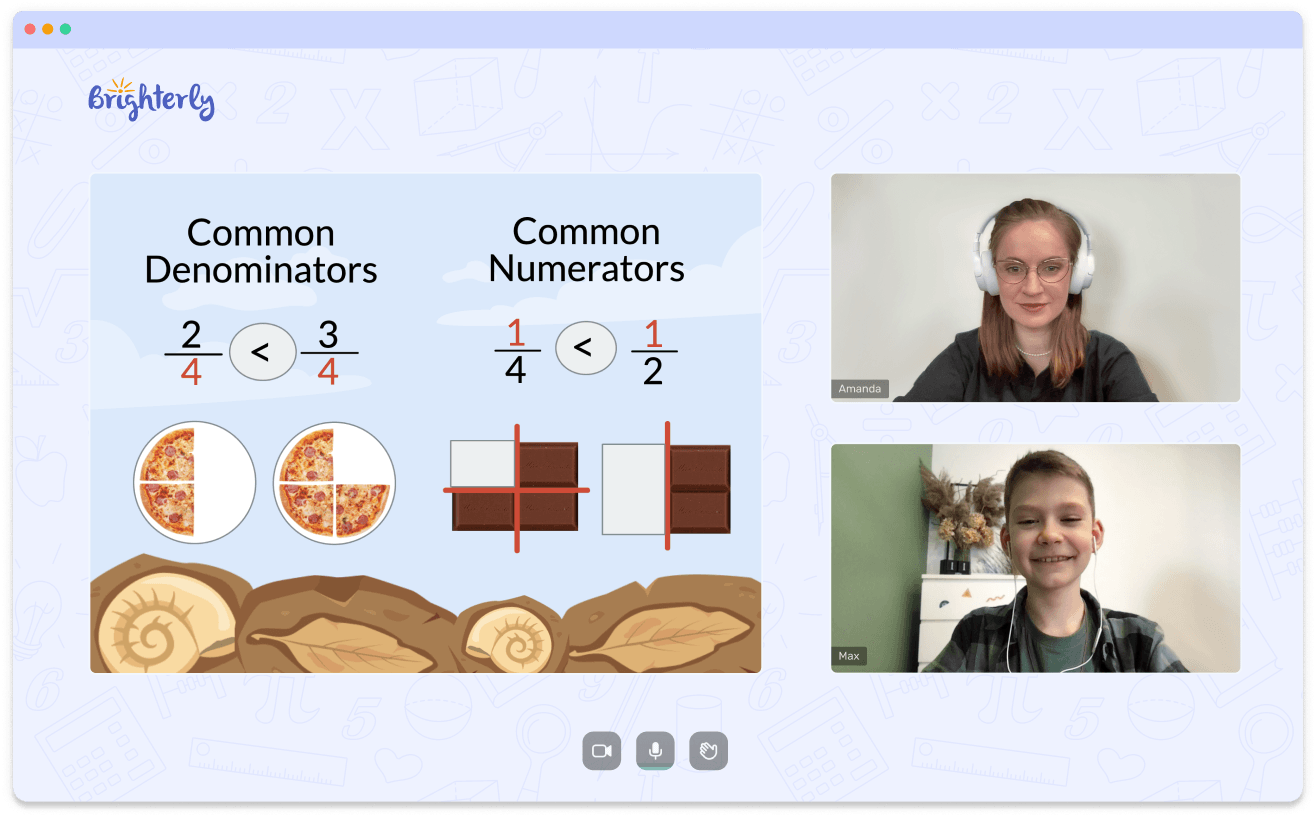# Triangle Congruence Worksheets

Congruent triangles may seem like a piece of cake on the surface. However, this isn’t always the case with young learners. For a student who’s just learning about congruence, it may take a while to get a hang of this concept. Fortunately, a congruent triangles worksheet is here to save the day. Designed for a curious young learner, it equips students with all the knowledge and skills they need to ace this topic. Let’s find out more about this worksheet, shall we?

## What Are Congruent Triangles?

Before we delve into the crux of the matter, it’s important to first establish what congruent triangles are. Put simply, congruent triangles are triangles that have the same size and shape. It means that the corresponding sides are equal. In the same vein, the corresponding angles have equal value too.

Leave your email and we will send you worksheets

worksheets sent successfully

Now, here’s the big question that often stumps young learners: how can you tell or prove that two triangles are congruent?

There are four rules that help us prove triangle congruence namely: the SSS rule, SAS rule, ASA rule, and AAS rule.

### The Side-Side-Side Rule

This rule states that if three sides of one triangle are equal to three sides of another triangle, then both triangles are congruent.

### The Side-Angle-Side Rule

The Side-Angle-Side rule is a tad different. According to this rule, if two sides and the included angle of one triangle are equal to two sides and the included angle of another triangle, then the triangles are congruent.

Math for Kids

Is Your Child Struggling With Math?
1:1 Online Math Tutoring### The Angle-Side-Angle Rule

According to the Angle-Side-Angle rule, if two angles and the included side of one triangle are equal to two angles and the included side of another triangle, then both triangles are congruent.

### The Angle-Angle-Side Rule

This rule states that if two angles and a non-included side of one triangle are equal to two angles and a non-included side of another triangle, then there is congruence.

## About the Proving Triangles Congruent Worksheet

This worksheet helps students learn more about triangle congruence and explains what this concept entails. It contains a wide range of exercises that are arranged in a progressive fashion, from simple to complicated. This approach will ease students into the concept, gradually building their skills as they ascend.

Leave your email and we will send you worksheets

worksheets sent successfully### Triangle Congruence Worksheets PDF

Triangle Congruence Worksheet### Triangle Congruence Worksheets PDF

Congruent Triangles Worksheet### Triangle Congruence Worksheets PDF

Proving Triangles Congruent Worksheet### Triangle Congruence Worksheets PDF

It also contains the answers to the exercises. Wondering why? Using triangle congruence worksheet answer keys, kids can have self-guided learning sessions where they cross-check what they’ve done against the answers. The congruent triangles worksheet answers aren’t cheat sheets. They’re simply guides.

## Benefits of the Triangle Congruence Worksheet Answers PDF

The triangle congruence worksheet is an excellent choice for young learners delving into this topic. These tools are interactive and loaded with sufficient visual stimulations to keep students engaged and ease the learning process. They will help a learner develop problem-solving skills and over time, master the concept of triangle congruence.

Most importantly, this worksheet is free!

### More Worksheets

Book 1 to 1 Demo Lesson• We help children understand Math concepts instead of memorizing.
• We will adapt to your child needs to improve math performance.

Is Your Child Struggling With Math?
Book 1 to 1 Demo Lesson